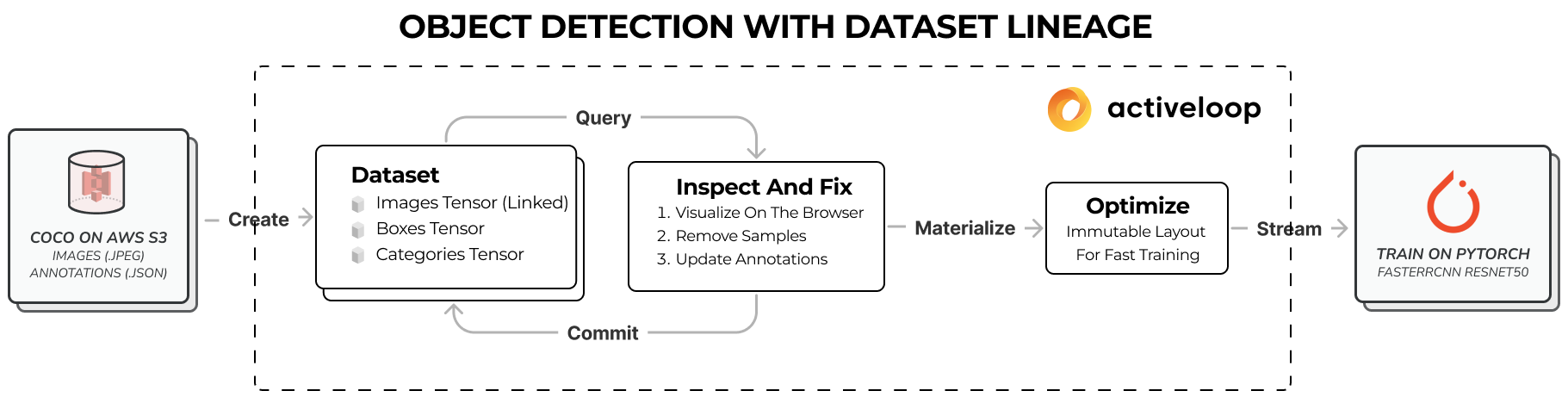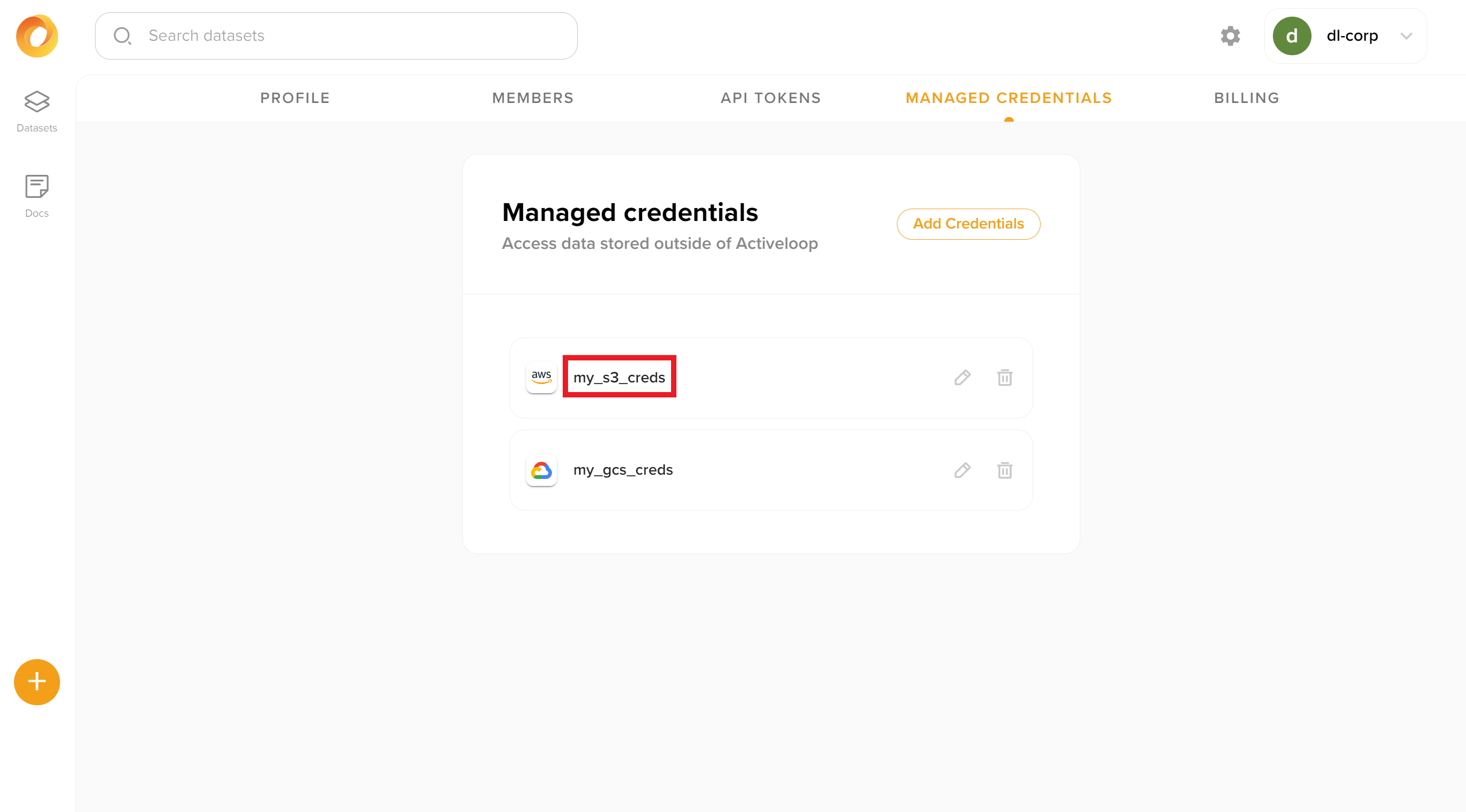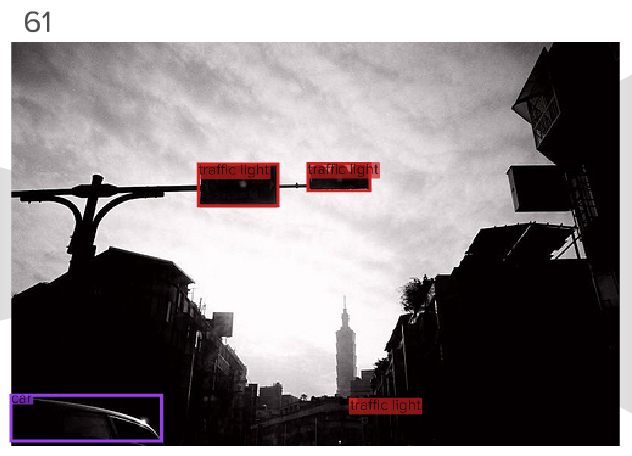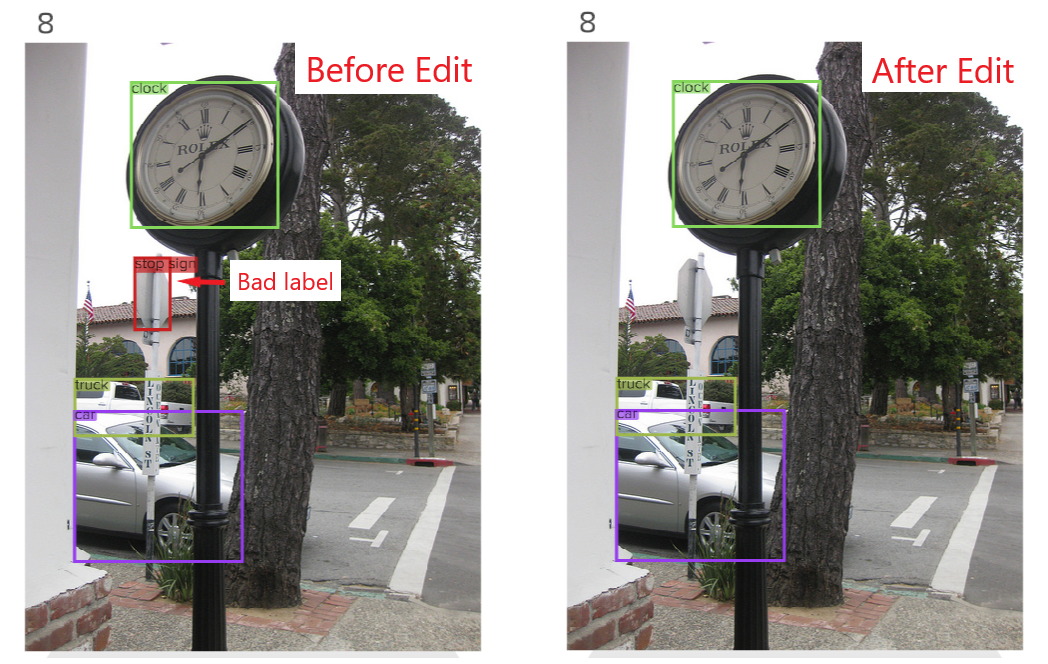Comment on page

# Querying, Training and Editing Datasets with Data Lineage

How to use queries and version control while training models.

## How to use queries and version control to train models with reproducible data lineage.

The road from raw data to a trainable deep-learning dataset can be treacherous, often involving multiple tools glued together with spaghetti code. Activeloop simplifies this journey so you can create high-quality datasets and train production-level deep-learning models.

#### This playbook demonstrates how to use Activeloop Deep Lake to:

• Create a Deep Lake dataset from data stored in an S3 bucket
• Visualize the data to gain insights about the underlying data challenges
• Update, edit, and store different versions of the data with reproducibility
• Query the data, save the query result, and materialize it for training a model.
• Train a object detection model while streaming data### Prerequisites

In addition to installation of commonly used packages, this playbook requires installation of:
pip3 install deeplake
pip3 install albumentations
pip3 install opencv-python-headless==4.1.2.30 #In order for Albumentations to work properly
pip install 'git+https://github.com/cocodataset/cocoapi.git#subdirectory=PythonAPI'
The required python imports are:
import deeplake
import numpy as np
import boto3
import math
import time
import os
from tqdm import tqdm
from pycocotools.coco import COCO
import albumentations as A
from albumentations.pytorch import ToTensorV2
import torch
import torchvision
from torchvision.models.detection.faster_rcnn import FastRCNNPredictor
You should also register with Activeloop and create an API token in the UI.

### Creating the Dataset

Since many real-world datasets use the COCO annotation format, the COCO training dataset is used in this playbook. To avoid data duplication, linked tensors are used to store references to the images in the Deep Lake dataset from the S3 bucket containing the original data. For simplicity, only the bounding box annotations are copied to the the Deep Lake dataset.
To convert the original dataset to Deep Lake format, let's establish a connection to the original data in S3.
dataset_bucket = 'non-hub-datasets'
s3 = boto3.resource('s3',
aws_access_key_id=os.environ.get('aws_access_key_id'),
aws_secret_access_key=os.environ.get('aws_secret_access_key'))
s3_bucket = s3.Bucket(dataset_bucket)
Next, let's load the annotations so we can access them later:
ann_path = 'coco/annotations/instances_train2017.json'
local_ann_path = 'anns_train.json'
coco = COCO(local_ann_path)
Moving on, let's create an empty Deep Lake dataset and pull managed credentials from Platform, so that we don't have to manually specify the credentials to access the `s3` links every time we use this dataset. Since the Deep Lake dataset is stored in Deep Lake storage, we also provide an API token to identify the user.
ds = deeplake.empty('hub://dl-corp/coco-train', token = 'Insert API Token')
creds_name = "my_s3_creds"
The UI for managed credentials in Platform is shown below, and more details are available here.Last but not least, let's create the Deep Lake dataset's tensors. In this example, we ignore the segmentations and keypoints from the COCO dataset, only uploading the bounding box annotations as well as their labels.
with ds:
ds.create_tensor('images', htype = 'link[image]', sample_compression = 'jpg')
ds.create_tensor('boxes', htype = 'bbox')
ds.create_tensor('categories', htype = 'class_label')
Finally, let's iterate through the data and append it to our Deep Lake dataset. Note that when appending data, we directly pass the s3 URL and the managed credentials key for accessing that URL using `deeplake.link(url, creds_key)`
with ds:
## ---- Iterate through each image and upload data ----- ##
for img_id in tqdm(img_ids):
#First create empty objects for all the annotations
boxes = np.zeros((len(anns),4))
categories = []
#Then populate the objects with the annotations data
for i, ann in enumerate(anns):
boxes[i,:] = ann['bbox']
categories.append([category_info[i]['name'] for i in range(len(category_info)) if category_info[i]['id']==ann['category_id']])
#If there are no categories present, append the empty list as None.
if categories == []: categories = None
img_url = "s3://{}/coco/train2017/{}".format(dataset_bucket, img_coco['file_name'])
"boxes": boxes.astype('float32'),
"categories": categories})
Note: if dataset creation speed is a priority, it can be accelerated using 2 options:
• By uploading the dataset in parallel. An example is available here.
• By setting the optional parameters below to `False`. In this case, the upload machine will not load any of the data before creating the dataset, thus speeding the upload by up to 100X. The parameters below are defaulted to `True` because they improve the query speed on image shapes and file metadata, and they also verify the integrity of the data before uploading. More information is available here:
verify = False,
create_shape_tensor = False,
create_sample_info_tensor = False
)

### Inspecting the Dataset

In this example, we will train an object detection model for driving applications. Therefore, we are interested in images containing cars, busses, trucks, bicycles, motorcycles, traffic lights, and stop signs, which we can find by running a SQL query on the dataset in Platform. More details on the query syntax are available here.
(select * where contains(categories, 'car') limit 1000)
union
(select * where contains(categories, 'bus') limit 1000)
union
(select * where contains(categories, 'truck') limit 1000)
union
(select * where contains(categories, 'bicycle') limit 1000)
union
(select * where contains(categories, 'motorcycle') limit 1000)
union
(select * where contains(categories, 'traffic light') limit 1000)
union
(select * where contains(categories, 'stop sign') limit 1000)
A quick visual inspection of the dataset reveals several problems with the data including:
• Sample `61` but is a-low quality image where it's very difficult to discern the features, and it is not clear whether the small object in the distance is an actual traffic light. Images like this do not positively contribute to model performance, so let's delete all the data in this sample.ds.pop(61)
ds.commit('Deleted index 61 because the image is low quality.')
• In sample `8`, a road sign is labeled as a `stop sign`, even though the sign is facing away from the camera. Even though it may be a `stop sign`, computer vision systems should positively identify the type of a road sign based on its visible text. Therefore, let's remove the stop sign label from this image.
ds.categories = ds.categories.numpy()[np.arange(0,4)!=2]
ds.boxes = ds.boxes.numpy()[np.arange(0,4)!=2,:]
ds.commit('Deleted bad label at index 8')Both changes are now evident in the visualizer, and they were both logged as separate commits in the version control history. A summary of this inspection workflow is shown below:

### Optimizing the Dataset for Training

Now that the dataset has been improved, we save the query result containing the samples of interest and optimize the data for training. Since query results are associated with a particular commit, they are immutable and can be retrieved at any point in time.
First, let's re-run the query and save the result as a dataset view, which is uniquely identified by an `id`.
The dataset is currently storing references to the images in S3, so the images are not rapidly streamable for training. Therefore, we materialize the query result (`Dataset View`) by copying and re-chunking the data for maximum performance:
ds.load_view('62d6d490e49d0d7bab4e251f', optimize = True, num_workers = 4)
Once we're finished using the materialized dataset view, we may choose to delete it using:
# ds.delete_view('62d6d490e49d0d7bab4e251f')

### Training an Object Detection Model

An object detection model can be trained using the same approach that is used for all Deep Lake datasets, with several examples in our tutorials. Typically the training would occur on another machine with more GPU power, so we start by loading the dataset and and corresponding dataset view:
ds = deeplake.load('hub://dl-corp/coco-train', token = 'Insert API Token')
When using subsets of datasets, it's advised to remap the input classes for model training. In this example, the source dataset has 81 classes, but we are only interested in 7 classes (cars, busses, trucks, bicycles, motorcycles, traffic lights, and stop signs). Therefore, we remap the classes of interest to values 0,1,2,3,4,6 before feeding them into the model for training. We also specify resolution for resizing the data before training the model.
WIDTH = 128
HEIGHT = 128
# These are the classes we care about and they will be remapped to 0,1,2,3,4,6 in the model
CLASSES_OF_INTEREST = ['car', 'truck', 'bus', 'motorcycle', 'bicycle', 'traffic light', 'stop sign']
# The classes of interest correspond to the following array values in the current dataset
INDS_OF_INTEREST = [ds.categories.info.class_names.index(item) for item in CLASSES_OF_INTEREST]
Next, let's specify an augmentation pipeline, which mostly utilizes Albumentations. We perform the remapping of the class labels inside the transformation function.
# Augmentation pipeline using Albumentations
tform_train = A.Compose([
A.RandomSizedBBoxSafeCrop(width=WIDTH, height=HEIGHT, erosion_rate=0.2),
A.Rotate(limit=20, p=0.5),
A.RandomBrightnessContrast(brightness_limit=0.1, contrast_limit=0.1, p=0.5),
A.Normalize(mean=(0.485, 0.456, 0.406), std=(0.229, 0.224, 0.225)),
ToTensorV2()
], bbox_params=A.BboxParams(format='pascal_voc', label_fields=['class_labels', 'bbox_ids'], min_area=16, min_visibility=0.6)) # 'label_fields' and 'box_ids' are all the fields that will be cut when a bounding box is cut.
# Transformation function for pre-processing the deeplake sample before sending it to the model
def transform_train(sample_in):
# Convert any grayscale images to RGB
image = sample_in['images']
shape = image.shape
if shape == 1:
image = np.repeat(image, int(3/shape), axis = 2)
# Convert boxes to Pascal VOC format
boxes = coco_2_pascal(sample_in['boxes'], shape)
# Filter only the labels that we care about for this training run
labels_all = sample_in['categories']
indices = [l for l, label in enumerate(labels_all) if label in INDS_OF_INTEREST]
labels_filtered = labels_all[indices]
labels_remapped = [INDS_OF_INTEREST.index(label) for label in labels_filtered]
boxes_filtered = boxes[indices,:]
# Make sure the number of labels and boxes is still the same after filtering
assert(len(labels_remapped)) == boxes_filtered.shape
# Pass all data to the Albumentations transformation
transformed = tform_train(image = image,
bboxes = boxes_filtered,
bbox_ids = np.arange(boxes_filtered.shape),
class_labels = labels_remapped,
)
# Convert boxes and labels from lists to torch tensors, because Albumentations does not do that automatically.
# Be very careful with rounding and casting to integers, becuase that can create bounding boxes with invalid dimensions
labels_torch = torch.tensor(transformed['class_labels'], dtype = torch.int64)
boxes_torch = torch.zeros((len(transformed['bboxes']), 4), dtype = torch.int64)
for b, box in enumerate(transformed['bboxes']):
boxes_torch[b,:] = torch.tensor(np.round(box))
# Put annotations in a separate object
target = {'labels': labels_torch, 'boxes': boxes_torch}
return transformed['image'], target
# Conversion script for bounding boxes from coco to Pascal VOC format
def coco_2_pascal(boxes, shape):
# Convert bounding boxes to Pascal VOC format and clip bounding boxes to make sure they have non-negative width and height
return np.stack((np.clip(boxes[:,0], 0, None), np.clip(boxes[:,1], 0, None), np.clip(boxes[:,0]+np.clip(boxes[:,2], 1, None), 0, shape), np.clip(boxes[:,1]+np.clip(boxes[:,3], 1, None), 0, shape)), axis = 1)
def collate_fn(batch):
return tuple(zip(*batch))
You can now create a PyTorch dataloader that connects the Deep Lake dataset to the PyTorch model using the provided method `ds_view.pytorch()`. This method automatically applies the transformation function and takes care of random shuffling (if desired). The `num_workers` parameter can be used to parallelize data preprocessing, which is critical for ensuring that preprocessing does not bottleneck the overall training workflow.
train_loader = ds_view.pytorch(num_workers = 8, shuffle = True,
transform = transform_train,
tensors = ['images', 'categories', 'boxes'],
batch_size = 16,
collate_fn = collate_fn)
This playbook uses a pre-trained torchvision neural network from the `torchvision.models` module. We define helper functions for loading the model and for training 1 epoch.
def get_model_object_detection(num_classes):
# Load an instance segmentation model pre-trained on COCO
model = torchvision.models.detection.fasterrcnn_resnet50_fpn(pretrained=True)
# Get number of input features for the classifier
# Replace the pre-trained head with a new one
return model
# Helper function for training for 1 epoch
model.train()
start_time = time.time()
images = list(image.to(device) for image in data)
targets = [{k: v.to(device) for k, v in t.items()} for t in data]
loss_dict = model(images, targets)
losses = sum(loss for loss in loss_dict.values())
loss_value = losses.item()
# Print performance statistics
if i%10 ==0:
batch_time = time.time()
speed = (i+1)/(batch_time-start_time)
print('[%5d] loss: %.3f, speed: %.2f' %
(i, loss_value, speed))
if not math.isfinite(loss_value):
print(f"Loss is {loss_value}, stopping training")
print(loss_dict)
break
losses.backward()
optimizer.step()
Training is performed on a GPU if possible. Otherwise, it's on a CPU.
device = torch.device('cuda') if torch.cuda.is_available() else torch.device('cpu')
print(device)
Let's initialize the model and optimizer.
model = get_model_object_detection(len(CLASSES_OF_INTEREST))
model.to(device)
# Specify the optimizer
params = [p for p in model.parameters() if p.requires_grad]
optimizer = torch.optim.SGD(params, lr=0.005,
momentum=0.9, weight_decay=0.0005)
The model and data are ready for training 🚀!
# Train the model for 1 epoch
num_epochs = 3
lr_scheduler = torch.optim.lr_scheduler.StepLR(optimizer, step_size=1, gamma=0.1)
for epoch in range(num_epochs): # Loop over the dataset multiple times
print("------------------ Training Epoch {} ------------------".format(epoch+1))